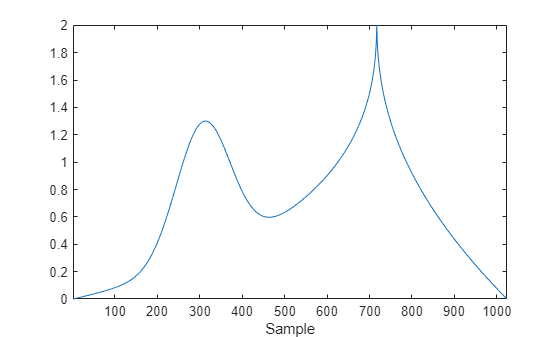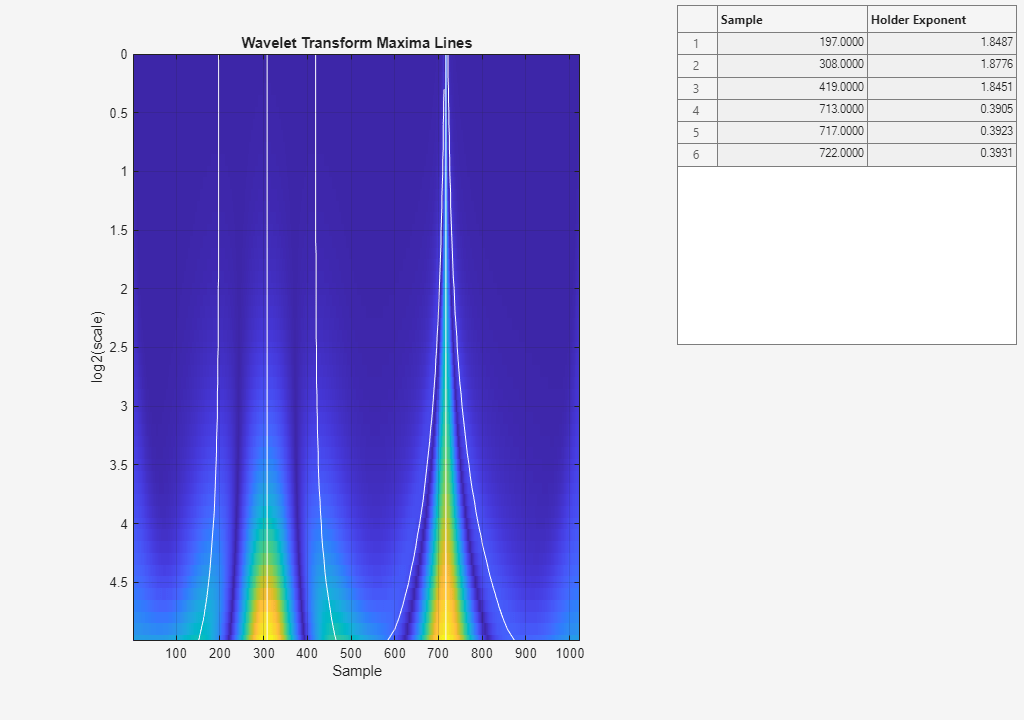localmax

Identify and chain local maxima

Syntax

[lmaxima,indices] = localmax(inputmatrix)
[lmaxima,indices] = localmax(inputmatrix,initrow)
[lmaxima,indices] = localmax(inputmatrix,initrow,regflag)

Description

[lmaxima,indices] = localmax(inputmatrix) identifies and chains the local maxima in the rows of inputmatrix.

[lmaxima,indices] = localmax(inputmatrix,initrow) initializes the chaining of local maxima beginning with row initrow. If there are no local maxima in initrow, all rows in lmaxima with indices less than initrow consist of only zeros.

[lmaxima,indices] = localmax(inputmatrix,initrow,regflag) replaces initrow of inputmatrix with the level-5 approximation (scaling) coefficients obtained with the sym4 wavelet.

Input Arguments

 inputmatrix inputmatrix is a matrix of real or complex numbers. Most often, inputmatrix is a matrix of continuous wavelet transform (CWT) coefficients, and you use localmax to identify maxima lines. localmax operates on the absolute values of inputmatrix. initrow Initialization row for chaining local maxima. The chaining algorithm begins at initrow and decrements the row index by 1 until the first row of the matrix is reached. By specifying initrow, you can exclude rows from the chaining algorithm. Default: size(inputmatrix,1) regflag Regularization flag. If you set regflag to true, the row of inputmatrix corresponding to initrow is replaced by the level-5 approximation (scaling) coefficients obtained with the sym4 wavelet. Default: true

Output Arguments

 lmaxima Matrix with local maxima chains. lmaxima only has nonzero entries at the locations of local maxima in the absolute values of inputmatrix. Denote the row index of lmaxima by R. You can determine the value of lmaxima at a local maximum in row R as follows: If R>initRow, the value of lmaxima at a local maximum is 1.If R=initRow, the value of lmaxima at a local maximum is the column index in row R.If R

Examples

Local Maxima of a Matrix

Construct a 4-by-4 matrix with local maxima at the following row-column indices: (4,2), (3,3), (2,2), and (1,3). Set initrow to 4 and regflag to false.

inputmatrix = ...
[3     2     5     3
4     6     3     2
4     4     7     4
4     6     2     2];
[lmaxima,indices] = localmax(inputmatrix,4,false);
lmaxima

Because localmax operates on the absolute values of inputmatrix, setting inputmatrix(4,2) = -inputmatrix(4,2) produces an identical lmaxima.

inputmatrix(4,2) = -inputmatrix(4,2);
[lmaxima1,indices1] = localmax(inputmatrix,4,false);
isequal(lmaxima,lmaxima1)

CWT Coefficient Moduli and Maxima Lines

Determine the local maxima from the CWT of the cuspamax signal using the default Morse wavelet. Plot the CWT coefficient moduli and maxima lines.

Plot the cuspamax signal and notice the shape of the signal near samples 300 and 700. The signal shows a cusp near sample 700.

plot(cuspamax);
xlabel('Sample');Plot the wavelet transform modulus maxima and note the local Holder exponent values at samples 308 and 717.

wtmm(cuspamax,'ScalingExponent','local');Holder exponent values indicate the strength of the singularities in a signal. Signal locations where the local Holder exponent is 0 are discontinuous at that location. Locations with Holder exponents greater than or equal to 1 are differentiable. Holder exponent values less than but close to 1 indicate that the signal at the location is almost differentiable. The closer the Holder exponent value is to 0, the stronger the singularity.

The Holder exponent at sample 308 is 1.9 and at sample 717 is 0.39. The low Holder value at sample 717 confirms that the signal is not differentiable and has a fairly strong singularity at that point.

Introduced in R2008a

Wavelet Toolbox DocumentationGet trial now